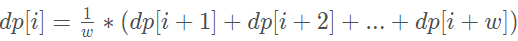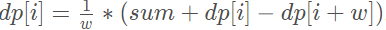## 题目描述

输入：N = 10, K = 1, W = 10



输入：N = 6, K = 1, W = 10



输入：N = 21, K = 17, W = 10



0 <= K <= N <= 10000
1 <= W <= 10000

## 解法

JAVA

class Solution {
public double new21Game(int N, int K, int W) {
if (K == 0) {
return 1.0;
}
double[] dp = new double[K + W+1];
double sum=0.0D;
for (int i = K; i <= N && i < K + W; i++) {
dp[i] = 1.0;
sum+=dp[i];
}

for (int i = K - 1; i >= 0; i--) {
dp[i] =sum/W;
sum=sum-dp[i+W]+dp[i];
}
return dp;
}
}


## 解题思路

### DP(动态规划）

• 抽取时，她从 [1, W] 的范围中随机获得一个整数作为分数进行累计
从1到w中随机取到一个数字，根据每个数字被取到的概率为1/W
• 爱丽丝以 0 分开始，并在她的得分少于 K 分时抽取数字
如果第一次抽的是1，第二次为2，以此类推，当抽取i次，手上的抽取的牌的综合为 sum=1+2+3+.....+i.，当sum>=K，停止抽取，如果还小于K，继续抽取从1到w中随机取到一个数字
• 爱丽丝的分数不超过 N 的概率是多少？
如果，第i次抽取时，总和sum>=k，停止抽取，如果sum<N，获胜，sum>N，则失败

dp[X]= (dp[X+1]+dp[X+2]~dp[X+W])/W；

• 如果X=K，停止抽牌，那么我牌总和最大为K-1，我最大抽取的牌为K-1+W,
• 当抽到了K，并且总和还小于N，那么我后面继续抽取K,K+N之间的胜率为100%，虽然题目要求不能抽取吗，那么K点的胜率是多少呢？
==>dp[K]=(dp[k]+dp[k+1]~dp[N]+0)/W；其中K<N;N<K+W
N以后的胜率为0不进行累计
double sum=0.0D;
//如果N的大于K;最多抽取K+W次，其中K~N的胜率都是1
for (int i = K; i <= N && i < K + W; i++) {
dp[i] = 1.0;
sum+=dp[i];
}


dp[K-1]= (dp[k-1+1]+dp[k-1++2]~dp[k-1+W])/W；

==>dp[K-1]=(dp[k]+dp[k+1]~dp[N]+0)/W；

=>sum/w；

sum+=dp[i]-dp[i+W];